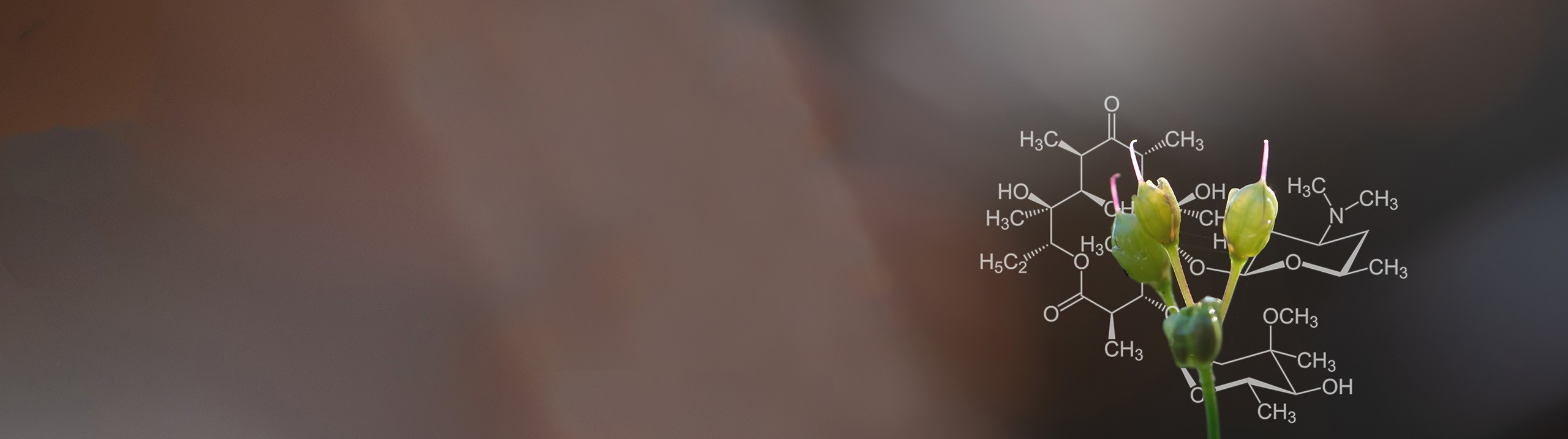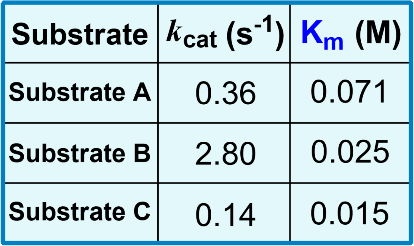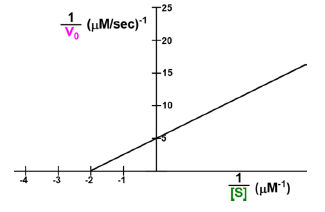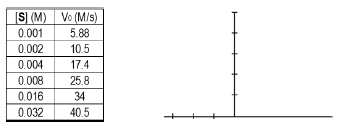Start typing, then use the up and down arrows to select an option from the list.# Biochemistry

Learn the toughest concepts covered in Biochemistry with step-by-step video tutorials and practice problems by world-class tutors

6. Enzymes and Enzyme Kinetics
1

#### concept

Specificity Constant3m
Play a video:
in this video, we're going to begin our discussions on the specificity constant oven enzyme. Now, before we talk directly about the specificity constant oven enzyme, it's first important to recognize a few key points. And the first key point to recognize is that when biochemists are studying or characterizing enzymes in a laboratory setting, it definitely is useful for them to study those enzymes in the lab under saturating substrate concentrations that are really, really high because they're able to determine value such as the V max and the K cat, or the maximal catalytic efficiency under saturating substrate concentrations. However, what's also really important to know is that the substrate concentrations within a cell or within a biological system are not always saturating. And so what this means is that saturating substrate concentrations is not always the best way to study enzymes. And again, this has to do with the fact that under physiological conditions or conditions within a cell again, the substrate concentrations are not always saturating. And instead the tendency is for the cell to maintain substrate concentrations approximately equal to the K M of the enzyme. Also another reason for why it's not always the best to study enzymes at saturating substrate concentrations is because under saturating substrate concentrations, that does not allow the biochemist to account for the binding affinity that an enzyme has for its substrate or the enzyme substrate. Binding affinity cannot be taken into account under saturating substrate concentrations, and that is because, regardless of the binding affinity that an enzyme has for substrate, even if the binding affinity is really, really, really low under saturating substrate concentrations, all of the enzyme is going to be associated with the substrate to form the enzyme substrate complex. And so again, that will not allow the biochemist to account for the binding affinity that enzyme has for its substrate under saturating substrate concentrations. And so again, what this really all means is that the maximal catalytic efficiency or the K cat that we talked about in some of our previous lesson videos, um, which Onley occurs under saturating substrate concentrations, is not always going to be the most relevant measure of the catalytic efficiency of an enzyme. And instead a better overall measure for the catalytic efficiency of an enzyme. Is the specificity constant? And so we're going to define and talk Maura about the specificity constant in our next lesson video. So I'll see you guys there
2

#### concept

Specificity Constant5m
Play a video:
So in our last lesson video, we said that the maximal catalytic efficiency of an enzyme indicated by the catalytic constant K cat or the turnover number is not always the best measure of catalytic efficiency, especially since it on Lee occurs under saturating substrate concentrations and saturating substrate. Concentrations are not always relevant, especially inside of a cell. And so, in this lesson video, we're going to talk about another, better overall measure of catalytic efficiency, specifically under non saturating substrate concentrations or low substrate concentrations. And that's exactly where the specificity constant comes into play. And that's because, as you guys may have already determined, the specificity constant is this ratio of the K cat to the K M. And that's exactly what we're saying. Down below is that the specificity constant is this ratio of the K cat over the K M. And so this specificity constant ratio of the kick out over the K M is really what determines an enzymes preference for a substrate specifically at non saturating substrate concentrations or low substrate concentrations. And so it turns out that substrate preference or the preference that an enzyme has for a particular substrate, is determined by the catalytic efficiency. And so the higher the catalytic efficiency, the greater the preference and enzyme has for that substrate. Now, it also turns out that catalytic efficiency actually depends on the concentration of substrate and whether or not those substrate concentrations are at saturating levels or non saturating levels. Now, if the substrate concentrations are at saturating levels, then that means that the catalytic efficiency is going to be determined by the maximal Catholic efficiency indicated by the K cat alone. And so, under saturating substrate concentrations, the Que cat alone is what dictates the preference that enzyme has for the substrate. However, when the substrate concentrations are at non saturating levels or low substrate concentrations, then the catalytic efficiency is actually determined by not the K cat alone, but instead the specificity constant, which is the ratio of the K cat over the K M. And that means that the specificity constant ratio is what determines the preference that an enzyme has for its substrate at non saturating or low substrate concentrations. Now, what I want you guys to recall from way back in our previous lesson videos is that we talked about this enzyme called Kimo trips and which is a pep today's that recognizes specific amino acids to cleave peptide bonds between them. And so what I want you guys to recall is that we said that kinda trips and has a preference for which amino acids it recognizes for cleavage and the pneumonic that helped us memorize that preference was free. Your worries like May, where we said that Kimo trips and has a preference for the aromatic amino acids, phenylalanine, tyrosine and trip to fan. And it has less of a preference for losing and Matheny. And so in our next video, we're going to talk about exactly how Kim a trip sends preference, relates directly to the specificity constant ratio. And so again, we're going to retouch on this idea here in our next lesson video. So hang on tight for this bullet point here. Now what I want you guys to note is that this ratio, the specificity, constant ratio of the cake cat over the K M is just another measure of catalytic efficiency and specifically the specificity. Constant ratio is the catalytic efficiency when an enzyme is not saturated with substrate or essentially at low substrate concentrations. Now this specificity. Constant ratio accounts for both the maximal catalytic efficiency K cat as well as an enzymes affinity for its substrate, which is indicated by the K M. Now, larger ratios of this specificity constant represent mawr efficient enzymes and, of course, more efficient. Enzymes, as we said earlier, is going to indicate a higher preference for the substrate at lower substrate concentrations. And I I also want to note that this specificity constant ratio of the cake cat over the K M actually does have a maximum value that's approximately equal to 10 to the ninth with units of inverse polarity, inverse seconds. And we're going to touch mawr on this idea later in our course. So also, hang on for this bullet point as well until later in our course. So this here concludes our introduction to the specificity constant ratio of the K cat over K M. And in our next video, we're going to be able to, uh, talk even mawr about the specificity, constant ratio and how it applies to Kimo Trip sins preference. And so I'll see you guys in that video
3

#### concept

Specificity Constant12m
Play a video:
4
Problem

Use the data in the chart below to provide answers to the following problems:

A) List the substrates from most preferred to least preferred under physiological conditions.

a) B, A, C.         b) C, B, A.         c) B, C, A.         d) A, C, B.B) List the substrates from most preferred to least preferred under saturating [S].

a) B, A, C.         b) C, B, A.         c) B, C, A.         d) A, C, B.

5

#### concept

Specificity Constant4m
Play a video:
So in our previous lesson, videos on specificity constant. We briefly mentioned that the specificity constant ratio of K cat over K M actually has a maximum value and it cannot be higher than that maximum value. And so it turns out that this limit to the maximum value of the specificity constant is actually diffusion controlled. And so the maximum value of this specificity constant of cake at over K M. Not only is it diffusion controlled limited, but it's also limited by the rate constant K one, which we know is the free enzyme and the free substrate association rate constant. And so this is because the free enzyme and the free substrate association will actually occur via diffusion. And so what this means is that the free enzyme and the free substrate can on Lee associate with each other to form the enzyme substrate complex as fast as the maximum rate of diffusion in the solvent. And of course, in biological systems, the solvent is going to be water. And so therefore, we can say that the maximum values of both the rate constant K one and the specificity constant of K cat over km are going to be equal to the maximum rate of diffusion in water, which is going to be 10 to the ninth. Inverse polarity, inverse seconds and so down below on the left hand side. Over here, what we have is our typical enzyme catalyzed reaction. And so all I want you guys to note is that the free enzyme and the free substrate can on Lee associate with each other via diffusion. And so what this means is that this rate constant here of K one is going to be limited by the maximum rate of diffusion. And so the K one value cannot be any greater than the maximum rate of diffusion. And also because we know from our previous lesson videos that the McHale is constant. K M is expressed as the ratio of the some of the enzyme substrate complex dissociation rate constants, or K minus one plus K two over the association rate constant K one. Because K one is included in the K M. What this means is that the K M is also going to be limited by the diffusion, uh, the max rate of diffusion and because the K M is included into the specificity constant, which is the ratio of the cake cat over the K. M. Uh, that means that the specificity constant is also going to be limited by diffusion. So up above notice. What we said is that the rate constant K 13 k m and the specificity constant of cake cat over cam are all directly limited by the maximum rate of diffusion in water, which again is 10 to the ninth. Inverse morality inverse seconds, just as we set up above. And so what's interesting to note here is that an enzyme whose, uh, specificity constant ratio is actually equal to this diffusion controlled max value that we indicated up above 10 to the ninth inverse similarity in verse seconds is actually referred to as a catalytic lee perfect enzyme. And so a catalytic lee perfect enzyme is essentially an enzyme who, upon the substrate binding to the enzyme, uh, the enzyme will immediately and almost instantaneously convert the substrate into product. And so that's why were we refer to these enzymes as Catalytic Lee Perfect. And so it's important to note here that the specificity constant ratio does have a maximum value, and it's going to be 10 to the ninth inverse similarity in verse seconds, and this maximum value is a diffusion controlled limit. And so this year concludes our lesson, and we'll be able to get some practice utilizing these concepts, moving forward in our practice problems, so I'll see you guys there.
6
Problem

Which of the following options is correct concerning the turnover number (k cat) and the specificity constant?

7
Problem

Use the Lineweaver-Burk plot to help you calculate the Vmax, kcat, Km and specificity constant for the enzyme.

Assume the [E]T = 2.9 nM. Hint: Pay close attention to units.Vmax = ___________.

kcat = ___________.

Km = ___________.

kcat / Km = ___________.

8
Problem

Explain the steps you could take to accurately find the K m, Vmax, and specificity constant for an enzyme from the following kinetic data, assuming the experiments were all done with [E]T = 0.1 mM.Step #1: ___________________________________________

Step #2: ___________________________________________

Step #3: ___________________________________________

Step #4: ___________________________________________

Step #5: ___________________________________________